Скачать презентацию Higher Dimensional Black Holes Tsvi Piran Evgeny Sorkin

82f56cfd50f2569f4727b79cf65c3d87.ppt

• Количество слайдов: 41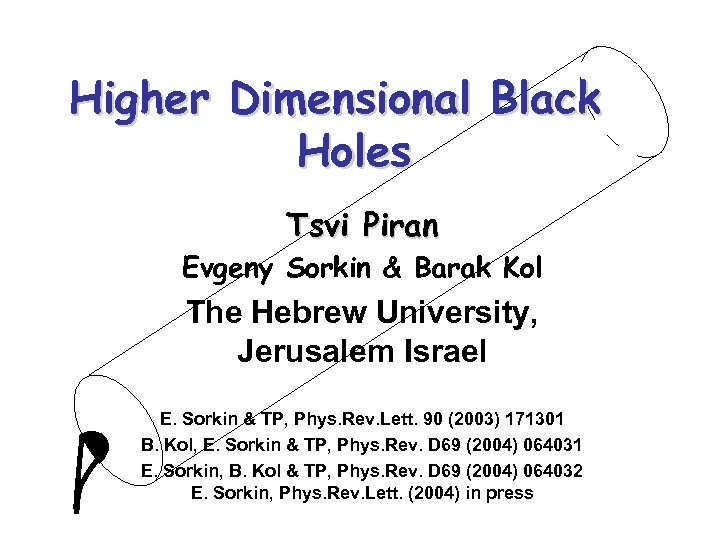Higher Dimensional Black Holes Tsvi Piran Evgeny Sorkin & Barak Kol The Hebrew University, Jerusalem Israel E. Sorkin & TP, Phys. Rev. Lett. 90 (2003) 171301 B. Kol, E. Sorkin & TP, Phys. Rev. D 69 (2004) 064031 E. Sorkin, B. Kol & TP, Phys. Rev. D 69 (2004) 064032 E. Sorkin, Phys. Rev. Lett. (2004) in press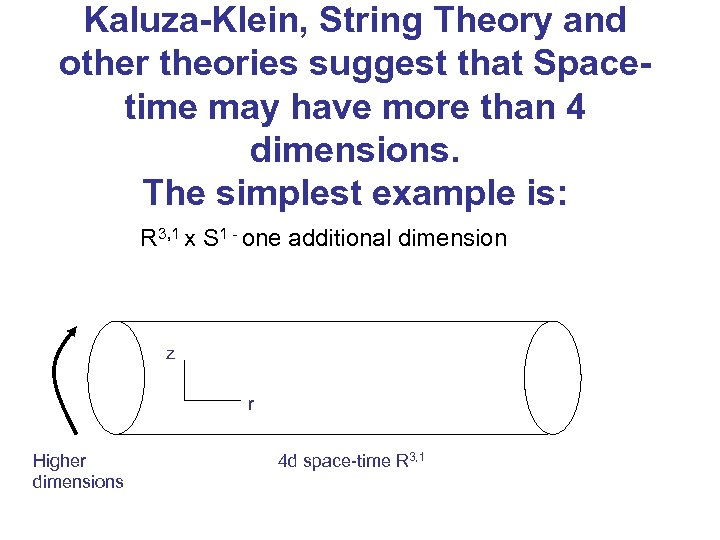Kaluza-Klein, String Theory and other theories suggest that Spacetime may have more than 4 dimensions. The simplest example is: R 3, 1 x S 1 - one additional dimension z r Higher dimensions 4 d space-time R 3, 1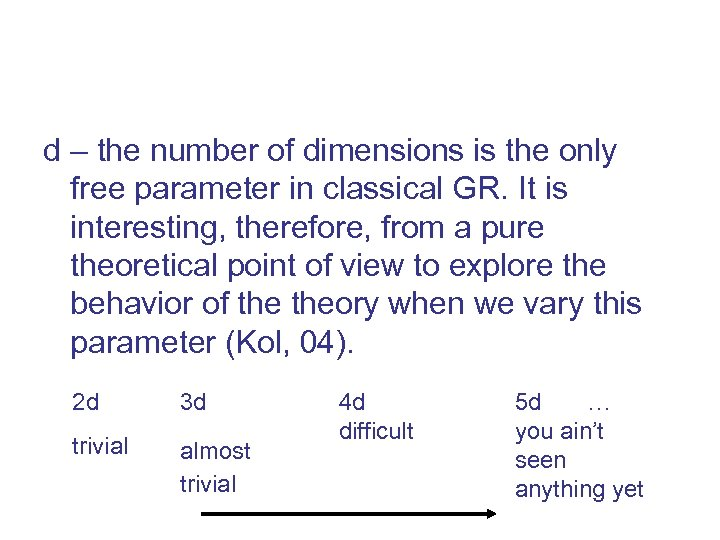d – the number of dimensions is the only free parameter in classical GR. It is interesting, therefore, from a pure theoretical point of view to explore the behavior of theory when we vary this parameter (Kol, 04). 2 d 3 d trivial almost trivial 4 d difficult 5 d … you ain’t seen anything yet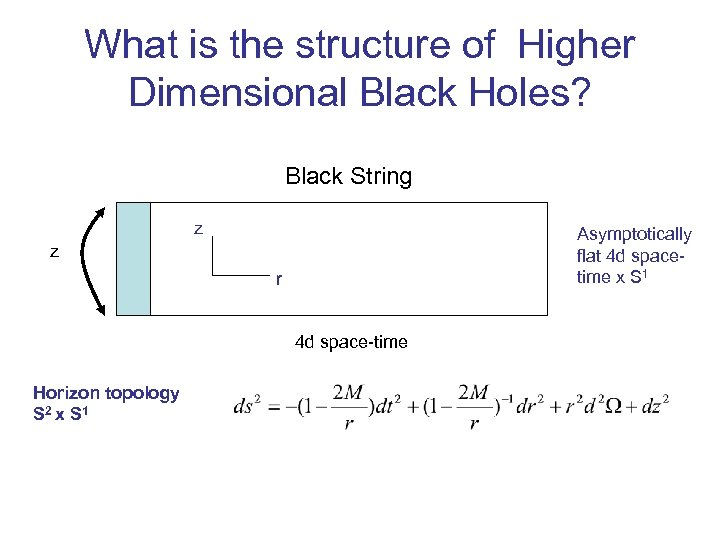What is the structure of Higher Dimensional Black Holes? Black String z Asymptotically flat 4 d spacetime x S 1 z r 4 d space-time Horizon topology S 2 x S 1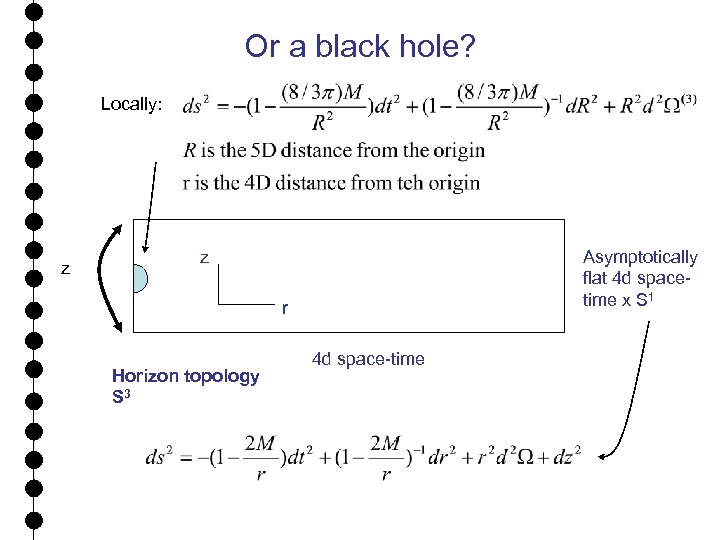Or a black hole? Locally: z z Asymptotically flat 4 d spacetime x S 1 r Horizon topology S 3 4 d space-time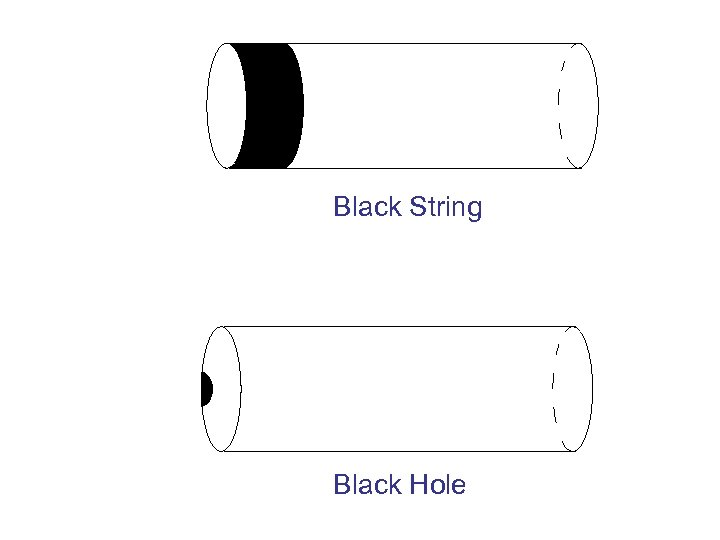Black String Black Hole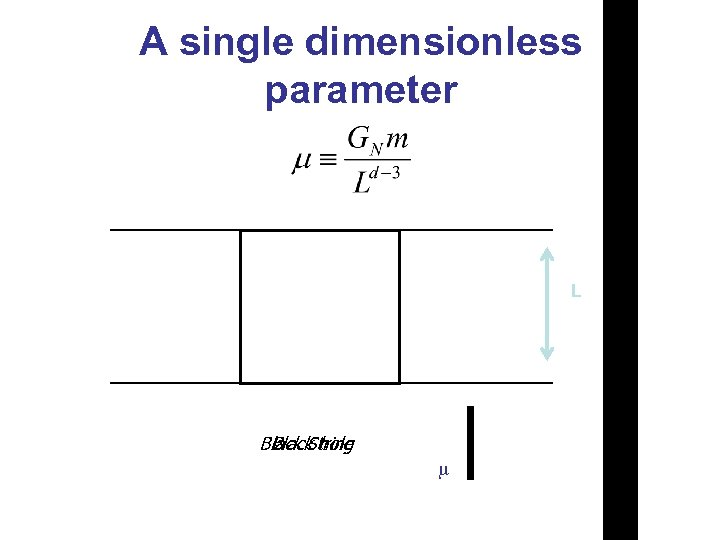A single dimensionless parameter L Black String Black hole m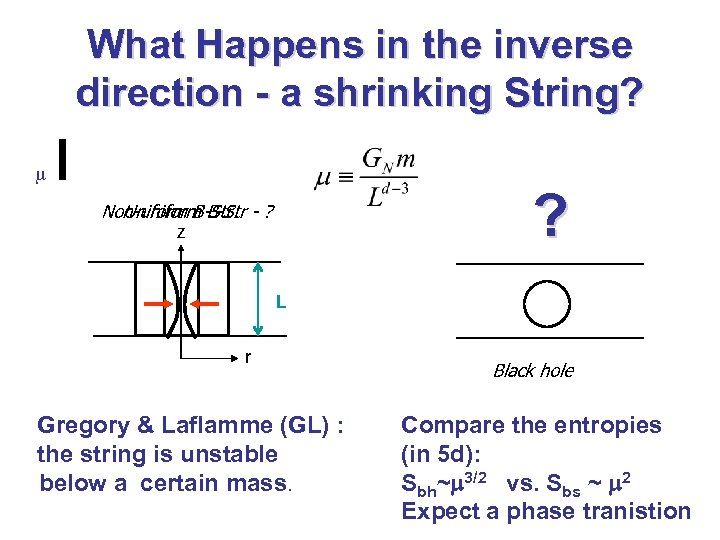What Happens in the inverse direction - a shrinking String? m ? Non-uniform B-Str - ? Uniform B-Str: z L r Gregory & Laflamme (GL) : the string is unstable below a certain mass. Black hole Compare the entropies (in 5 d): Sbh~m 3/2 vs. Sbs ~ m 2 Expect a phase tranistion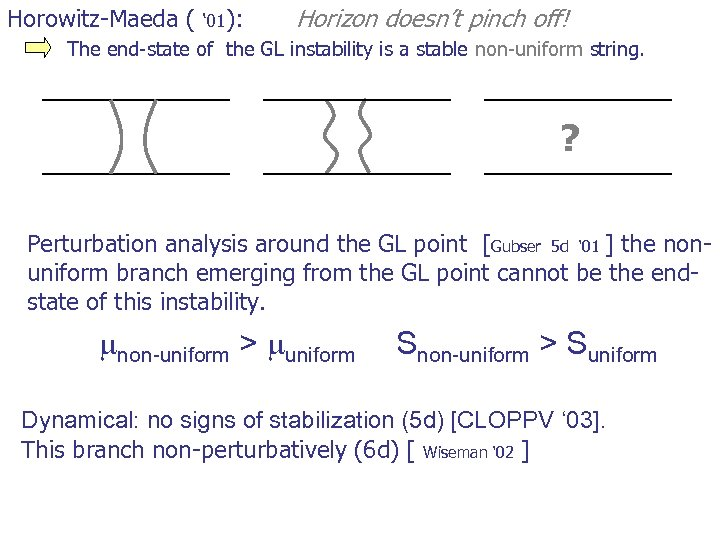Horowitz-Maeda ( ‘ 01): Horizon doesn’t pinch off! The end-state of the GL instability is a stable non-uniform string. ? Perturbation analysis around the GL point [Gubser 5 d ‘ 01 ] the nonuniform branch emerging from the GL point cannot be the endstate of this instability. mnon-uniform > muniform Snon-uniform > Suniform Dynamical: no signs of stabilization (5 d) [CLOPPV ‘ 03]. This branch non-perturbatively (6 d) [ Wiseman ‘ 02 ]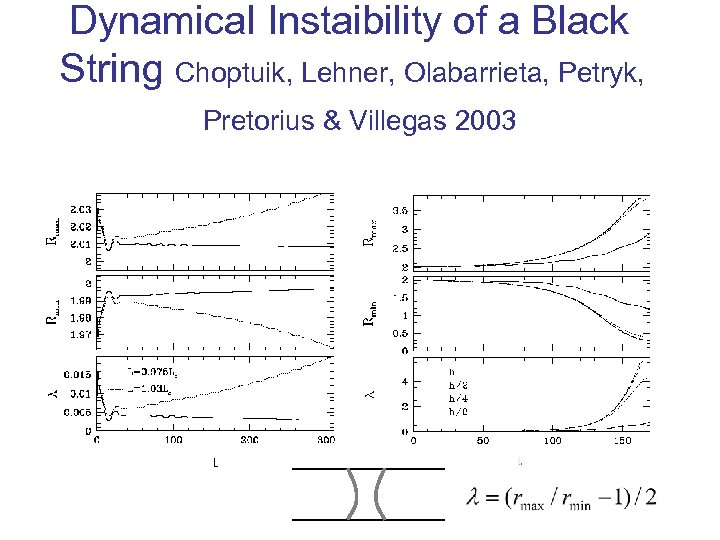Dynamical Instaibility of a Black String Choptuik, Lehner, Olabarrieta, Petryk, Pretorius & Villegas 2003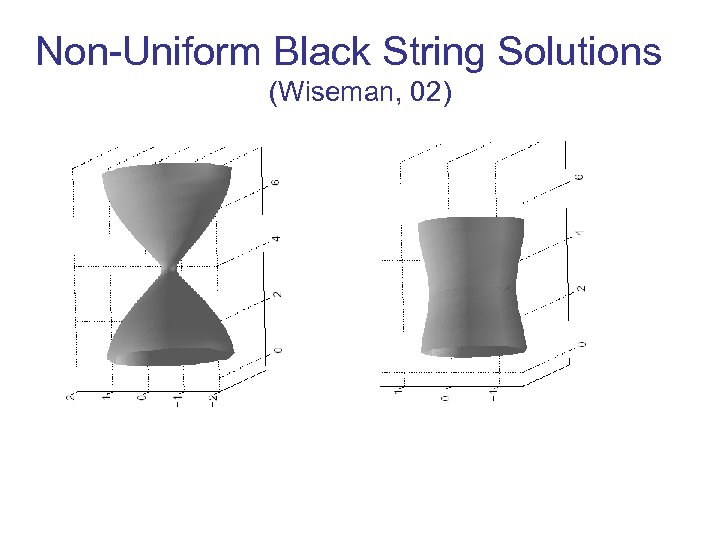Non-Uniform Black String Solutions (Wiseman, 02)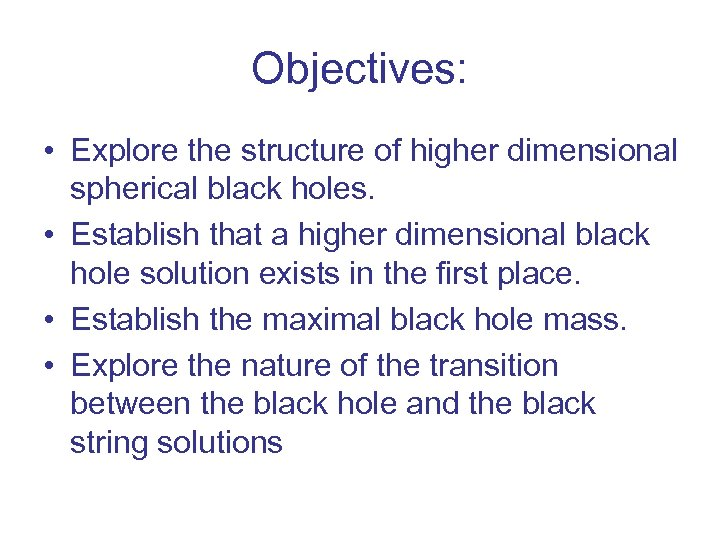Objectives: • Explore the structure of higher dimensional spherical black holes. • Establish that a higher dimensional black hole solution exists in the first place. • Establish the maximal black hole mass. • Explore the nature of the transition between the black hole and the black string solutions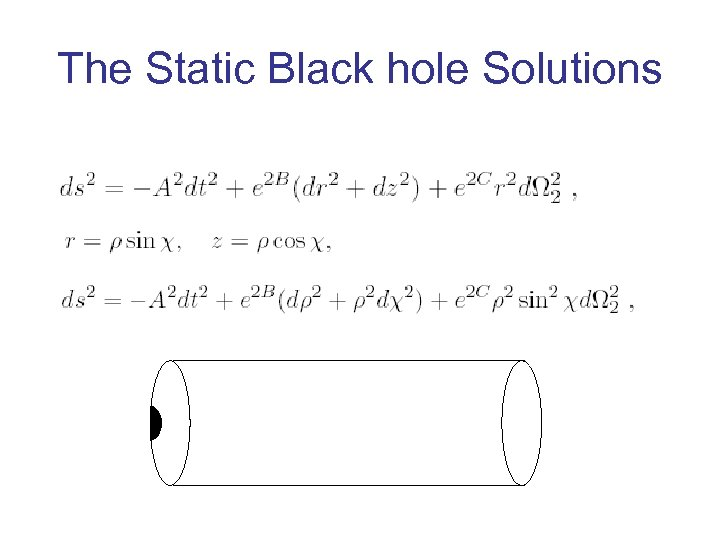The Static Black hole Solutions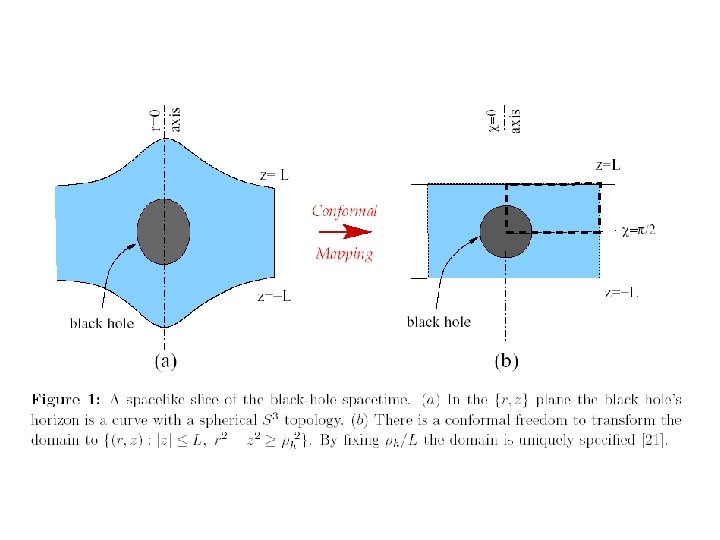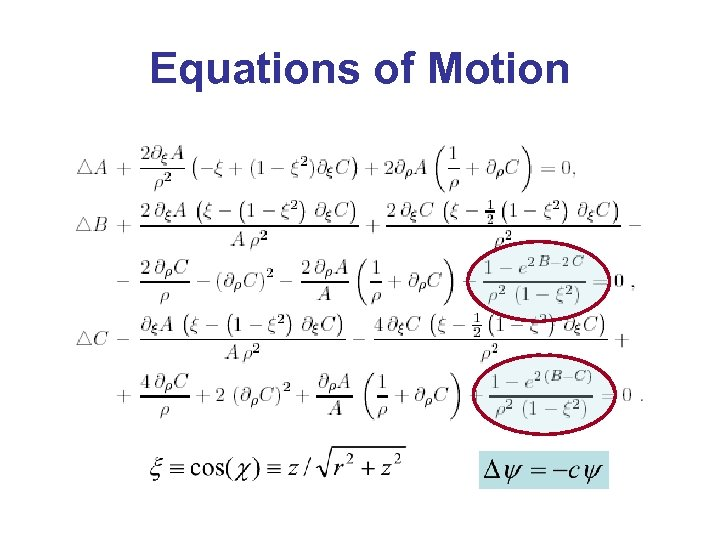Equations of Motion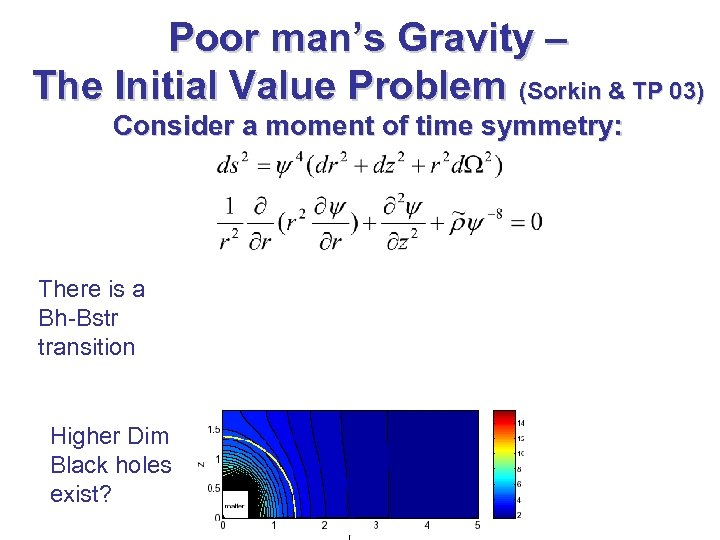Poor man’s Gravity – The Initial Value Problem (Sorkin & TP 03) Consider a moment of time symmetry: There is a Bh-Bstr transition Higher Dim Black holes exist?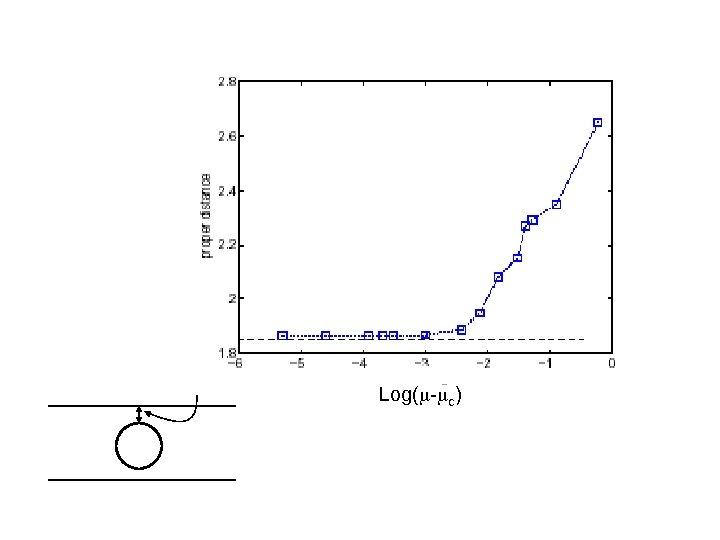Log(m-mc) Proper distance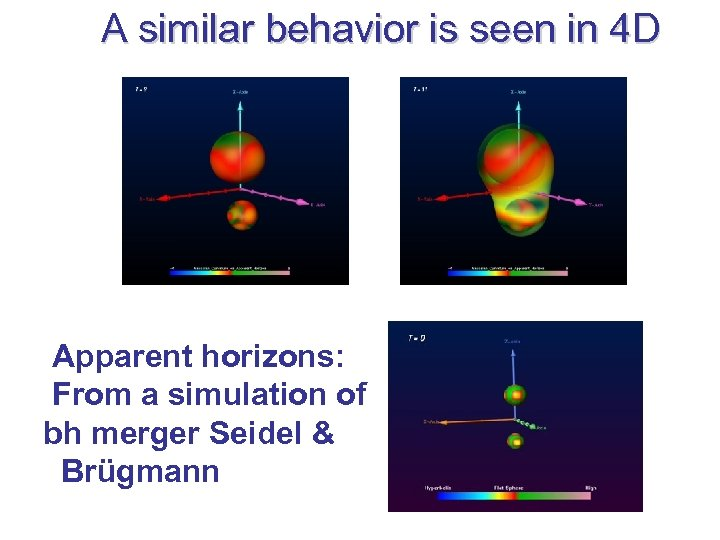A similar behavior is seen in 4 D Apparent horizons: From a simulation of bh merger Seidel & Brügmann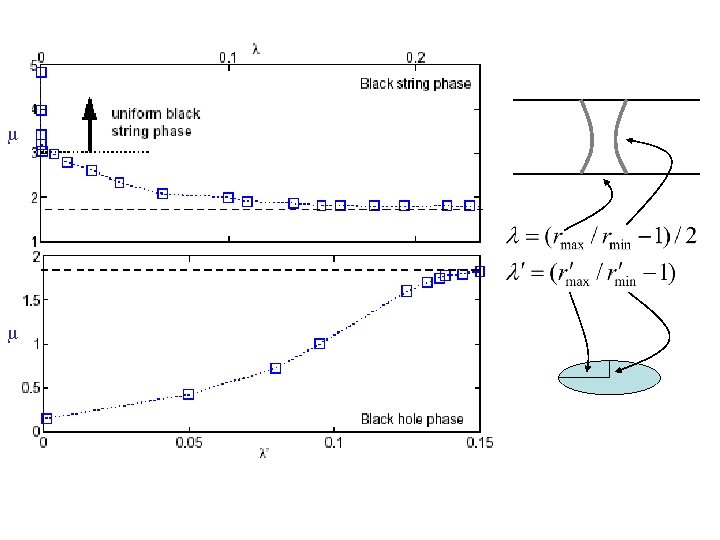m m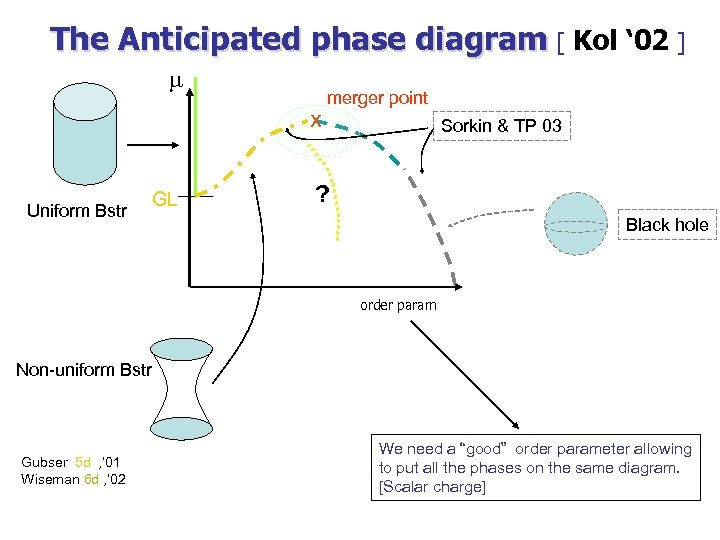The Anticipated phase diagram [ Kol ‘ 02 ] m x Uniform Bstr GL merger point Sorkin & TP 03 ? Black hole order param Non-uniform Bstr Gubser 5 d , ’ 01 Wiseman 6 d , ’ 02 We need a “good” order parameter allowing to put all the phases on the same diagram. [Scalar charge]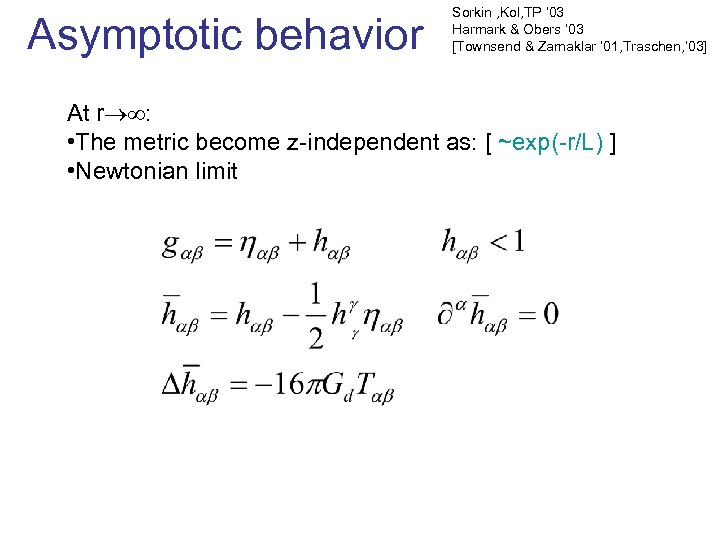Asymptotic behavior Sorkin , Kol, TP ‘ 03 Harmark & Obers ‘ 03 [Townsend & Zamaklar ’ 01, Traschen, ’ 03] At r : • The metric become z-independent as: [ ~exp(-r/L) ] • Newtonian limit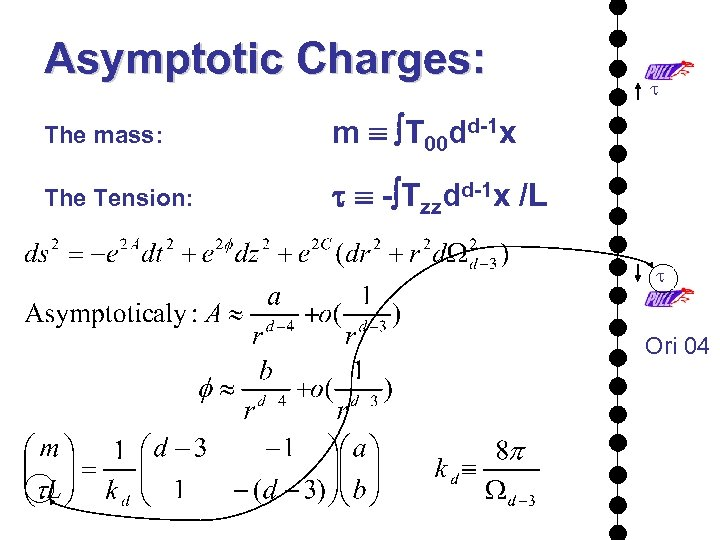Asymptotic Charges: The mass: m T 00 dd-1 x The Tension: t t - Tzzdd-1 x /L t Ori 04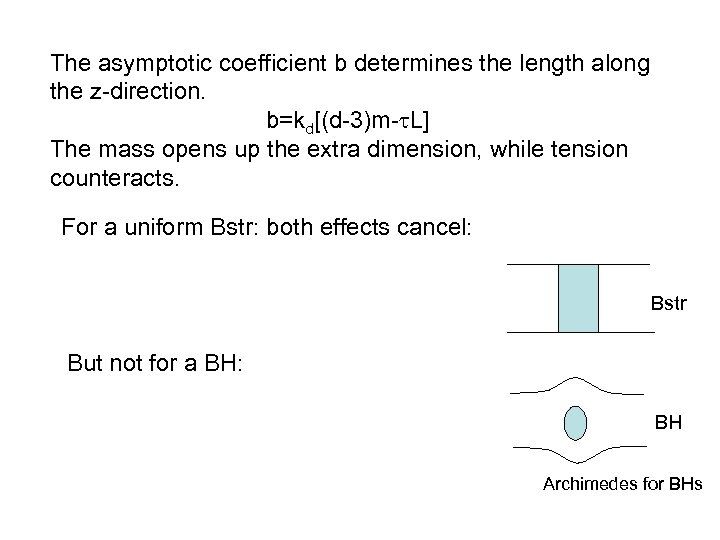The asymptotic coefficient b determines the length along the z-direction. b=kd[(d-3)m-t. L] The mass opens up the extra dimension, while tension counteracts. For a uniform Bstr: both effects cancel: Bstr But not for a BH: BH Archimedes for BHs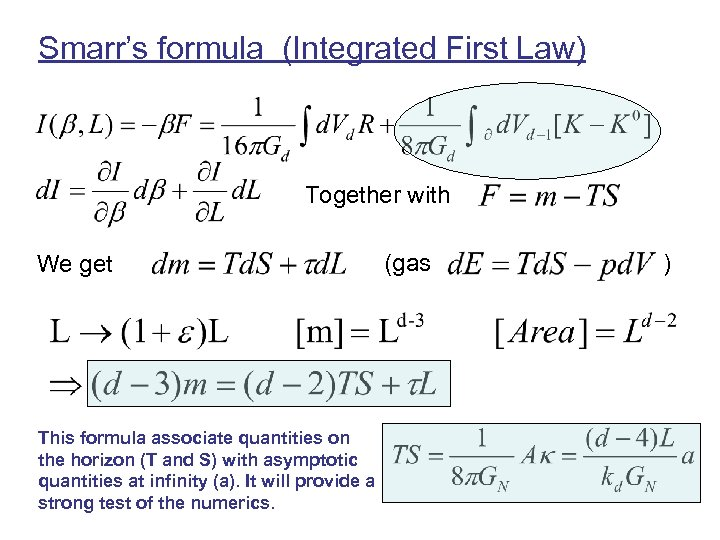Smarr’s formula (Integrated First Law) Together with We get This formula associate quantities on the horizon (T and S) with asymptotic quantities at infinity (a). It will provide a strong test of the numerics. (gas )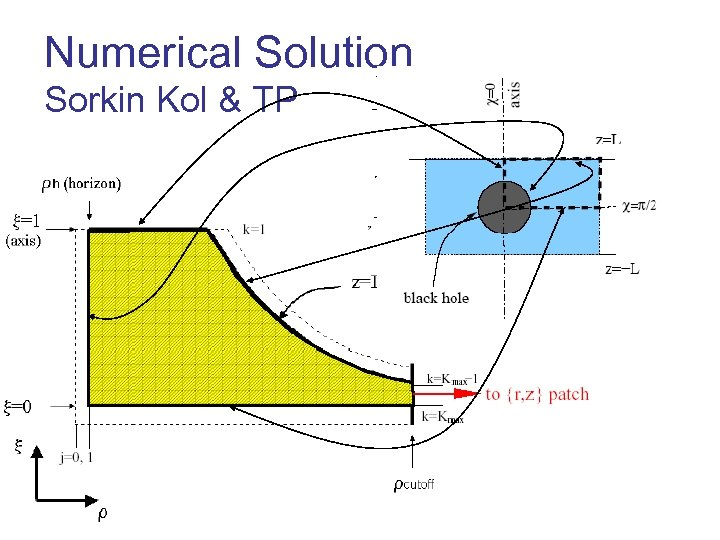Numerical Solution Sorkin Kol & TP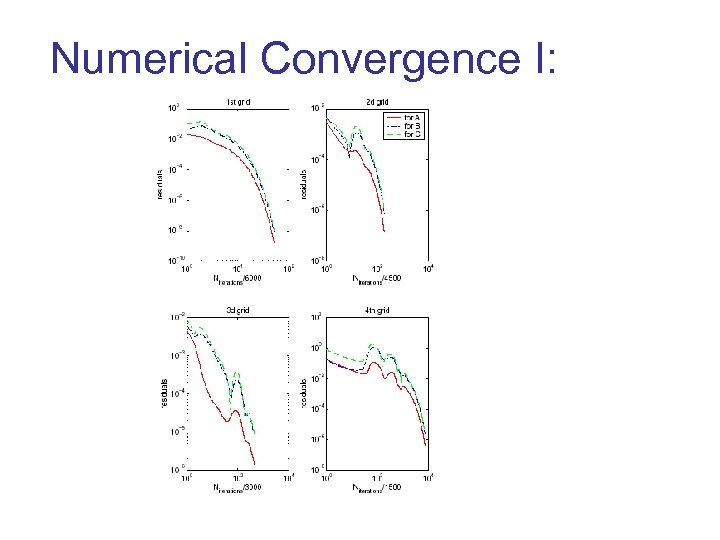Numerical Convergence I: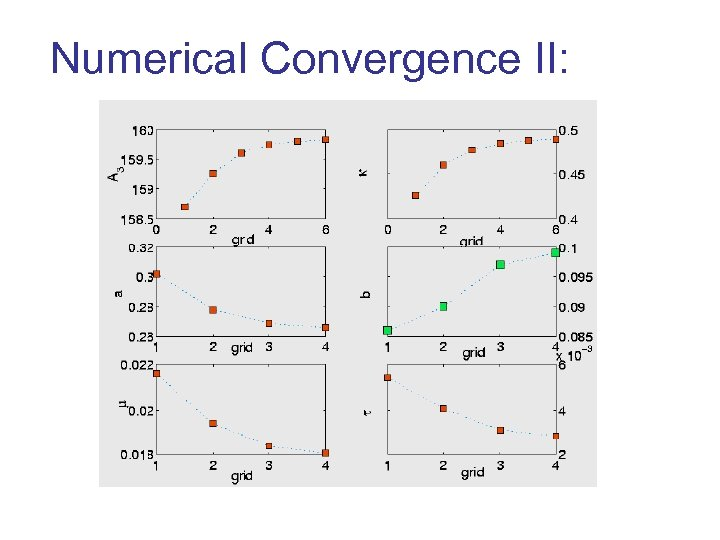Numerical Convergence II: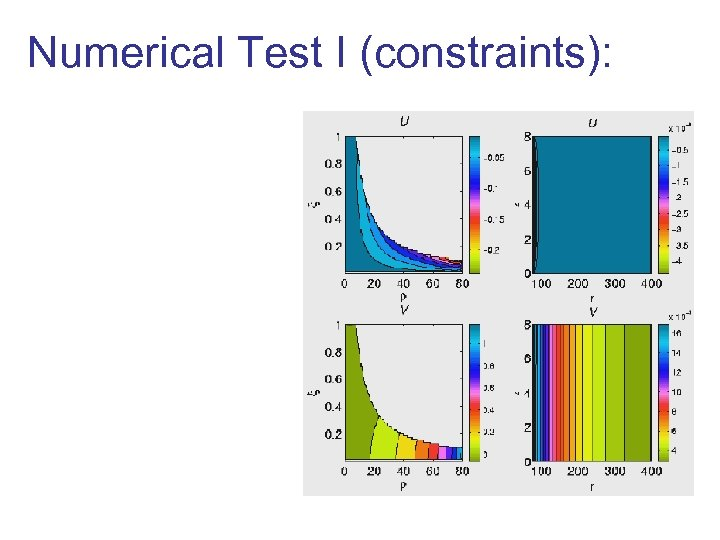Numerical Test I (constraints):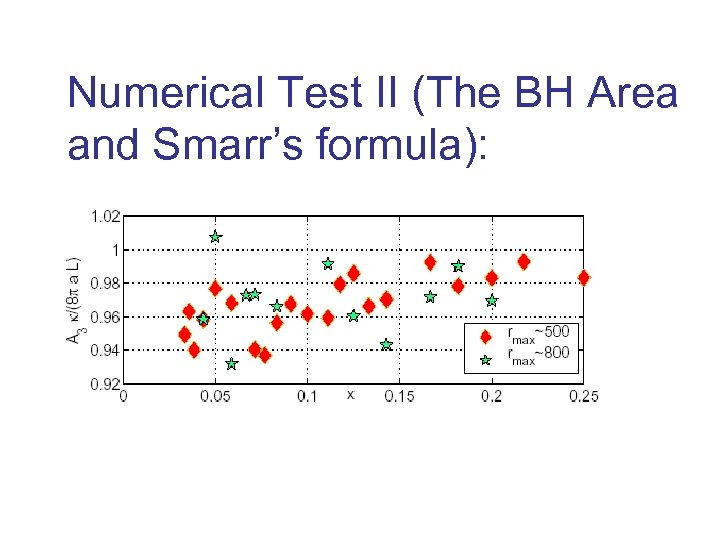Numerical Test II (The BH Area and Smarr’s formula):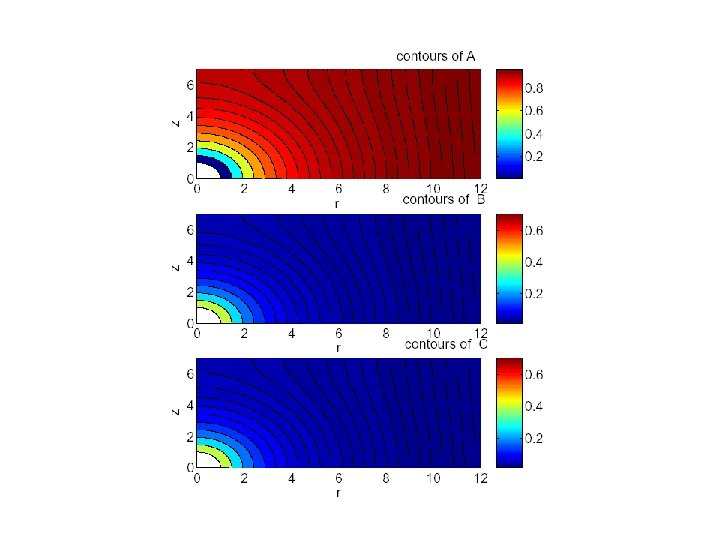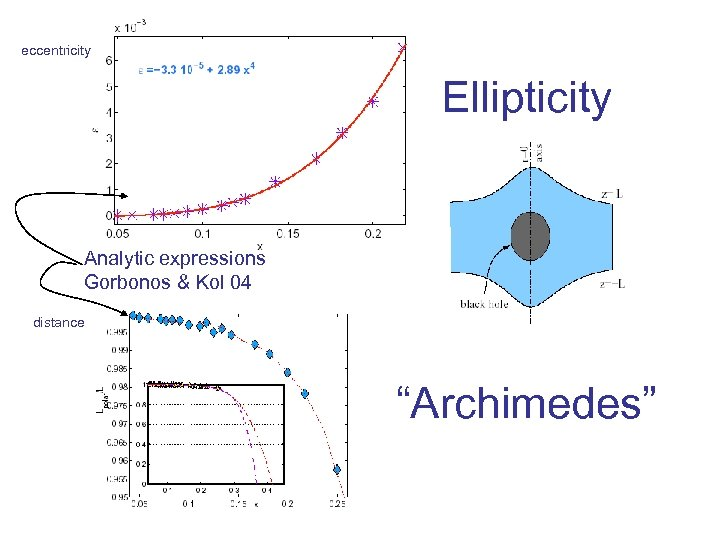eccentricity Ellipticity Analytic expressions Gorbonos & Kol 04 distance “Archimedes”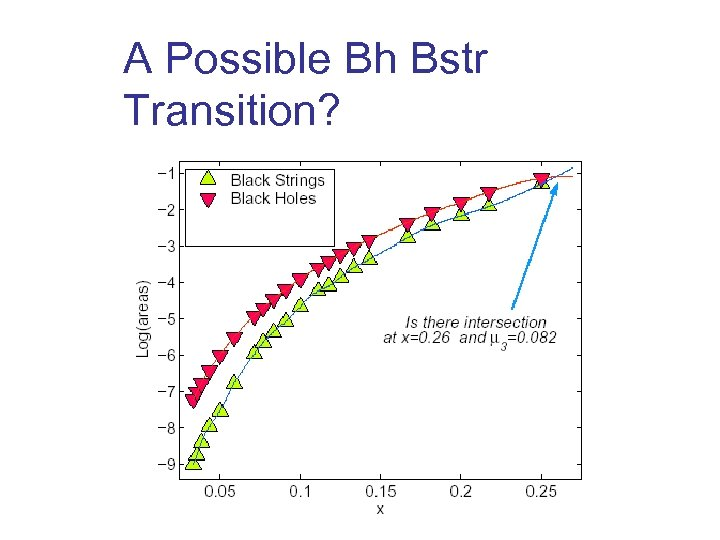A Possible Bh Bstr Transition?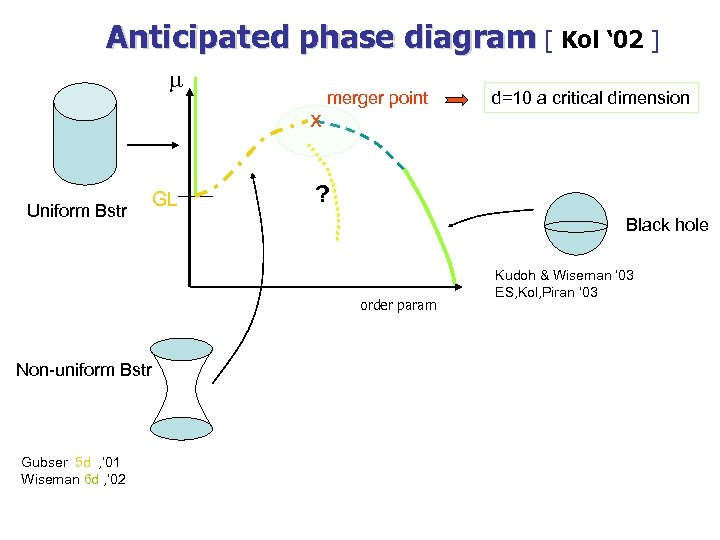Anticipated phase diagram [ Kol ‘ 02 ] m x Uniform Bstr GL merger point ? Black hole order param Non-uniform Bstr Gubser 5 d , ’ 01 Wiseman 6 d , ’ 02 d=10 a critical dimension Kudoh & Wiseman ‘ 03 ES, Kol, Piran ‘ 03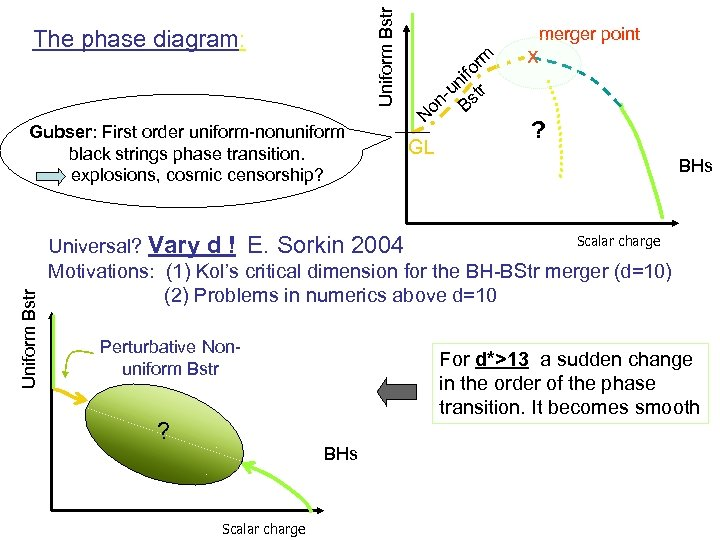Uniform Bstr The phase diagram: Uniform Bstr Gubser: First order uniform-nonuniform black strings phase transition. explosions, cosmic censorship? merger point rm fo i un tr n- Bs o N GL x ? BHs Scalar charge Universal? Vary d ! E. Sorkin 2004 Motivations: (1) Kol’s critical dimension for the BH-BStr merger (d=10) (2) Problems in numerics above d=10 Perturbative Nonuniform Bstr ? For d*>13 a sudden change in the order of the phase transition. It becomes smooth BHs Scalar charge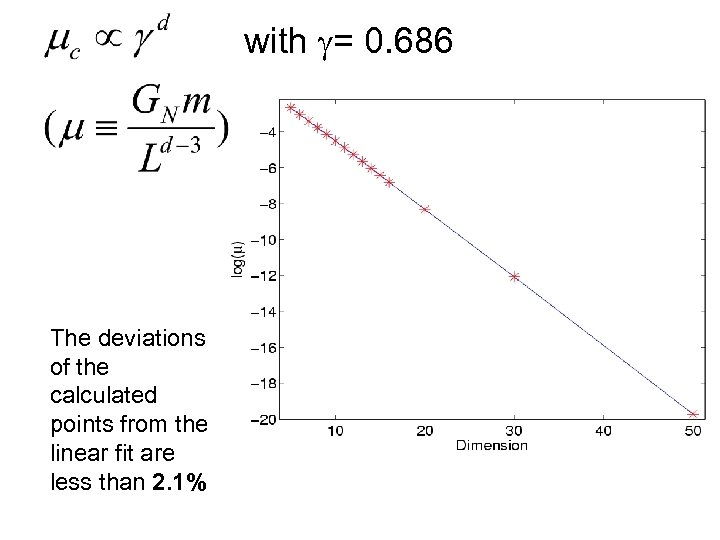with g= 0. 686 The deviations of the calculated points from the linear fit are less than 2. 1%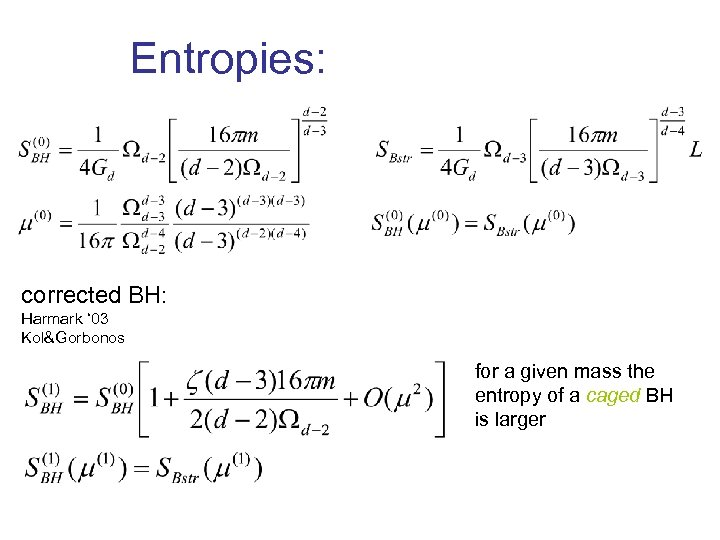Entropies: corrected BH: Harmark ‘ 03 Kol&Gorbonos for a given mass the entropy of a caged BH is larger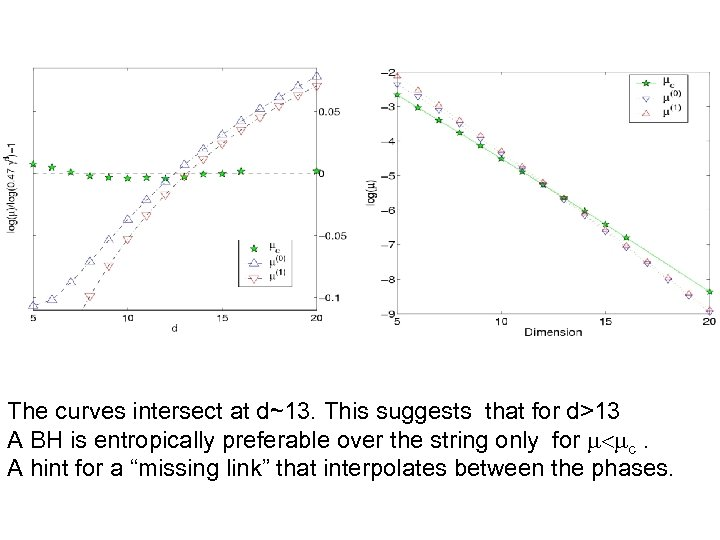The curves intersect at d~13. This suggests that for d>13 A BH is entropically preferable over the string only for m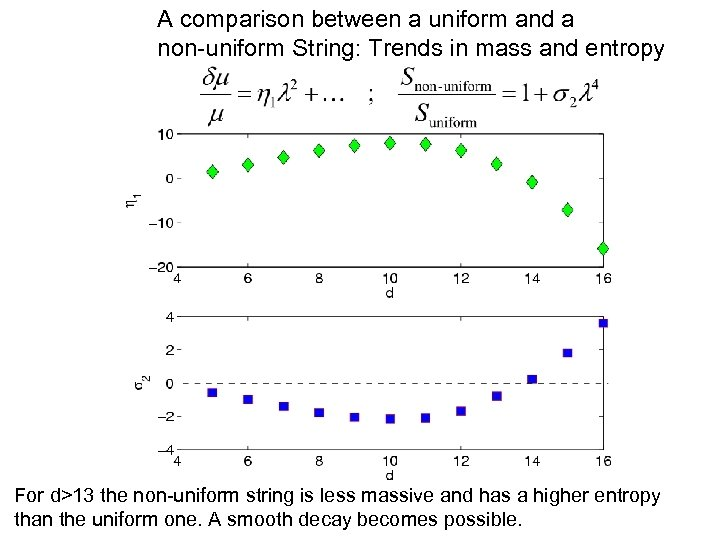A comparison between a uniform and a non-uniform String: Trends in mass and entropy For d>13 the non-uniform string is less massive and has a higher entropy than the uniform one. A smooth decay becomes possible.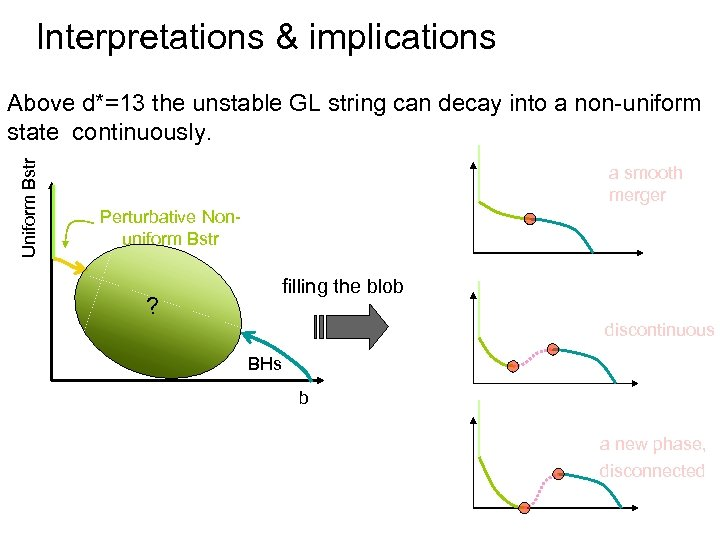Interpretations & implications Uniform Bstr Above d*=13 the unstable GL string can decay into a non-uniform state continuously. a smooth merger Perturbative Nonuniform Bstr filling the blob ? discontinuous BHs b a new phase, disconnected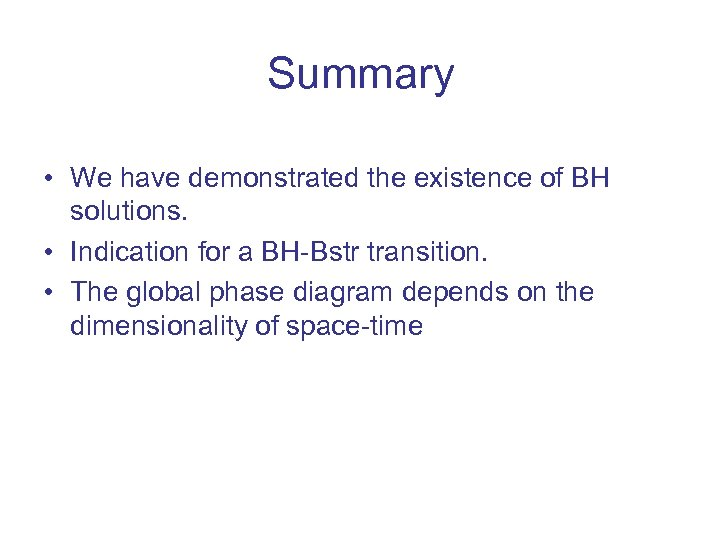Summary • We have demonstrated the existence of BH solutions. • Indication for a BH-Bstr transition. • The global phase diagram depends on the dimensionality of space-time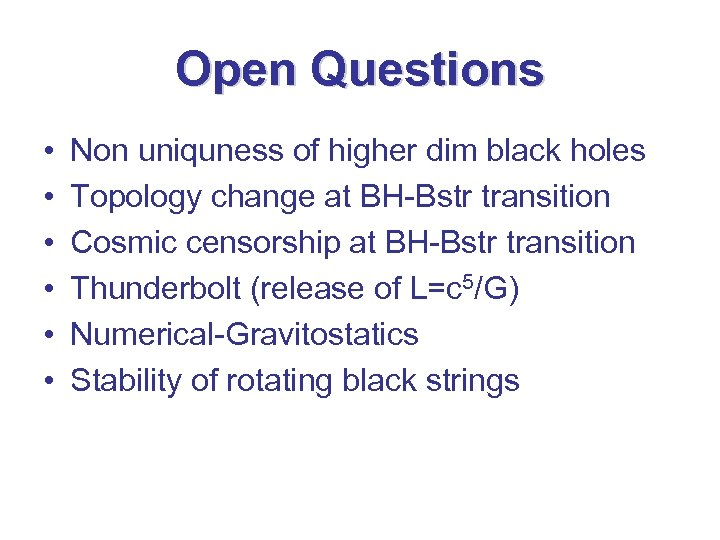Open Questions • • • Non uniquness of higher dim black holes Topology change at BH-Bstr transition Cosmic censorship at BH-Bstr transition Thunderbolt (release of L=c 5/G) Numerical-Gravitostatics Stability of rotating black strings